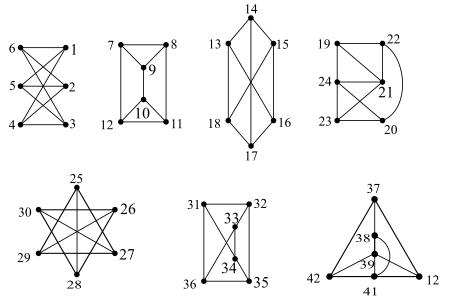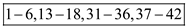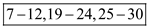Problem

# Solutions For Applied Combinatorics Chapter 1.2 Problem 7E

#### Step-by-Step Solution

Solution 1

Isomorphic Graph: A graph that may be in different forms but having the same number of vertices, edges, and also the same edge connectivity. Two graphs G and H are called isomorphic if:

a. Their number of components that is vertices and edges are same.

b. Their edge connectivity is retained.

Consider the graphs which are provided in the textbook problem as shown below,The objective is to find the pairs of graphs in above set are isomorphic.

All above graphs have same number of vertices and edges but different number of edge connectivity.

First, third, sixth and seventh have same number of vertices, edges and edge connectivity. So, this set is isomorphic.

And, second, fourth and fifth have same number of vertices, edges and edge connectivity.

So, this set is another set of isomorphic graphs.

Hence, Graphsandare mutually isomorphic.